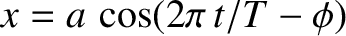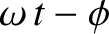## Creating electromagnetic waves: the LC circuit

I have consulted this resource for writing the following text.

Let us consider an electric circuit that is composed of a capacitor and an inductor. Let us charge the capacitor using a battery in an accessory circuit (as shown in the first image of the above site). This will be removed after charging. Let us consider that in this figure we have just charged the capacitor and that phenomena are ready to start.

We know that an electric current consists of electrons moving. However in physics, there is a specific convention: the direction of the current is considered to be that of the positive charges. In this figure, charges that repel each other between the two plates of the capacitor start moving. The electric current is symbolized by i and has the direction of positive charges. The energy that is stored as potential between the plates of the capacitor becomes kinetic energy of the electrons that move.

As the electric current flows in the inductor, it creates a magnetic field with the direction shown on the figure that will tend to create a voltage that will resist to the increase of the current (Lenz law). As the charges on the plates of the capacitor become less, electrons will not push each other with force to move in the circuit and the current will be decreasing.

The inductor had been creating a stronger and stronger magnetic field and in this way it was storing the kinetic energy of the electrons in its magnetic field. As the current decreases, it will tend to oppose this decrease and will create a voltage that will try to enhance the current. Under this influence,  the capacitor will start charging in the opposite direction. E.g. we shall see positive charges in the opposite plate. When all the energy stored in the inductor will be depleted then the capacitor will be fully charged and the current will be zero. This is depicted in the image below.

As positive and negative charges at the opposite sites of the capacitor will repel themselves, the capacitor will start discharging and a current of the opposite direction will run in the circuit. Notice that the magnetic field of the inductor has changed direction.

This will continue until the capacitor has discharged. The inductor will keep the current going and the capacitor will start to charge in the opposite direction. Eventually, the capacitor will be charged and the current will be zero. We will notice that the capacitator will be charged with the same polarity as shown in the beginning.

Let us represent the phenomenon graphically using a blue line for charge and a red dashed line for current in the graph below.

The charge of the capacitor decreases, becomes zero, increases (capacitor charges with opposite polarity); then decreases, becomes zero, and increases to reach the initial value (polarity same as beginning).

We notice that the phenomenon repeats itself in a certain period of time. In other words, the phenomenon is periodical and is characterized by a period. We know that the frequency is the inverse of the period. E.g. by considering the mechanical waves section we could ask: “How frequent are the ups and down of the green bead?" If the green bead does 2 ups and down per second, then the period of the phenomenon, or one up and down is 0.5 second.

As we see in the figure, the current and the charge at the capacitor present a sinusoidal change with time and the same applies for the voltage of  the capacitor & inductor and the energies stored in these elements.

We have seen in the mechanical waves sections how these ups and down are linked to an angle, an angle that “runs” with a specific let us say “angular velocity” which, is termed “angular frequency". It is an angle that does 360 degrees in one period; or 6.28 rads (2π rads) in a period. Therefore: ω=2π/Τ.

It is possible to find the period or the ω of the phenomenon?

I am coping below some information from this link:

According to Kirchhoff's second circuital law, the sum of the potential drops across the various components of a closed circuital loop is equal to zero (Grant and Philips 1975). In other words,(33)

Dividing by L, and differentiating with respect to t, we obtain(34)

where(35)

This is the natural angular frequency of the circuit. It characterises the behavior of the moving electrical charges in the circuit. As mentioned in a previous section, moving electrical charges correspond to an electromagnetic field. This circuit generates an electromagnetic signal with an angular frequency ω.

Here is an example from the following link:

In the above figure, suppose C = 250nF and initially charged, and L = 4.0μH .  Find (a) the natural angular frequency w , (b) the natural frequency f, and (c) the period T of the oscillations.

Solution:  (a) w = 1 / SQRT( LC ) =  1 / [(250x10-9F)(4.0x10-6H)] = 1.0x106 rd/s

(b) w = 2π f    ;    f = w/2π    ;    f  = 1.0x106 / 2π  =  160,000 Hz  or 160 kHz

(c) T = 1 / f    ;     T = 1 / 160,000s-1  =  6.28x10-6 seconds.

___________________________________________________________________________

Note that the LC circuit actually generates an alternating current (AC) as shown by the red dashed line in the figure above.

Here is some information on the AC we use in our electric grid.

https://en.wikipedia.org/wiki/Utility_frequency

The utility frequency, (power) line frequency (American English) or mains frequency (British English) is the frequency of the oscillations of alternating current (AC) in an electric power grid transmitted from a power plant to the end-user. In large parts of the world this is 50 Hz, although in the Americas and parts of Asia it is typically 60 Hz.

(...) places that now use the 50 Hz frequency tend to use 220–240 V, and those that now use 60 Hz tend to use 100–127 V.

How can we transmit an electromagnetic signal?

Let us take this signal and send it away or in other words transmit it. We will use an antenna for this purpose. We wouldn't want to interefere in this circuit by connecting something. We know that Faraday had discovered that if we approach another circuit then electricity will be induced to it. We will therefore take another inductor so as to replicate fully the magnetic phenomena generated by the inductor of the LC circuit and connect it to an antenna as shown in the figure below.

What is an antenna and how does it work exactly?

https://en.wikipedia.org/wiki/Dipole_antenna

The simplest and most widely used class of antenna is the dipole antenna which consists of two identical conductive elements that are billaterally symmetrical. The current from the LC circuit is applied at the center. Electrons are pushed back and forth, charging the ends of the antena positively and negatively in an alternating fashion. This creates electromagnetic waves that propagate in space with the speed of light.Here is an animation from  https://en.wikipedia.org/wiki/Antenna_(radio)Animation of a half-wave dipole antenna transmitting radio waves. Showing electric field lines.

How can we capture an electromagnetic signal e.g. a cell phone signal?Let us say that this is a cell phone signal of 2 GHz coming on to the antenna. How can we capture it?

The answer is that we have to make our LC circuit tune-in or resonate with that frequency. In other words its natural angular frequency must be the same so as to achieve resonance. We saw above that there exists a specific relation between ω and the components of the LC circuits.

Here is an example from the following link:

The frequency used for communications in cell phones is about 2000MHz or 2.0GHz.  Find (a) the angular frequency for such signals.  (b) If in the receiver a 0.050pF-capacitor is used, find the inductance L of the inductor to be used to create resonance at that frequency.

Solution:  (a) w = 2π f    ;    w = 2π(2.0x109 Hz) = 1.256x1010 rd/s  or (1.3E10)rd/s

(b) w = 1 / SQRT( LC )    ;    w2 = 1 / LC    ;    L = 1 / [Cw2]   =   1.27x10-7 Henry

As mentioned at the same link, if we want to capture a specific radio station or frequency e.g. France Culture 93.5MHz we have to "adjust the LC circuit to have the natural frequency we want; in other words, we can tune the receiver (the radio set) to the desired station with this simple circuit.  The tuning knob on most radio sets changes the capacity of that variable capacitor."

(A capacitor whose capacity can be changed is used).

From wikipedia

 Light comparison Name Frequency (Hz) (Wavelength) Photon energy (eV) Gamma ray > 30 EHz (0.01 nm) 124 keV - 300+ GeV X-Ray 30 EHz - 30 PHz (0.01 nm - 10 nm) 124 eV to 120 keV Ultraviolet 30 PHz - 750 THz (10 nm - 400 nm) 3.1 eV to 124 eV Visible 750 THz - 428.5 THz (400 nm - 700 nm) 1.7 eV - 3.1 eV Infrared 428.5 THz - 300 GHz (700 nm - 1 mm) 1.24 meV - 1.7 eV Microwave 300 GHz - 300 MHz (1 mm - 1 m) 1.24 µeV - 1.24 meV Radio 300 MHz - 3 kHz (1 m - 100 km) 12.4 feV - 1.24 meV

Interesting forum post

https://www.quora.com/How-do-you-create-electromagnetic-waves-and-manipulate-its-frequencies

How do you create electromagnetic waves and manipulate its frequencies?